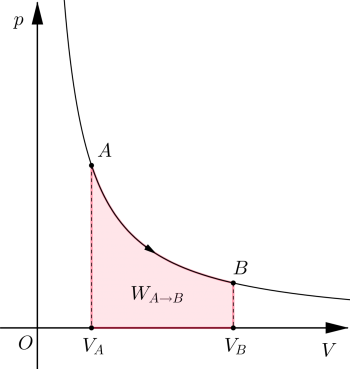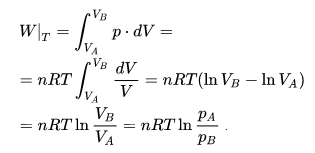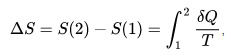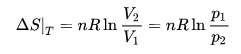Isothermal ProcessIn thermodynamics, an isothermal process is a thermodynamic transformation at constant temperature, that is, a variation of the state of a physical system during which the temperature of the system does not change with time. Devices called thermostats can maintain a constant temperature value.

The isothermal transformation of a perfect gas is described by Boyle's law which, in a pressure-volume diagram (or Clapeyron's plane), is represented by a branch of the equilateral hyperbola.

Isotherm of a perfect gas

Calculation of heat and work exchanged

For isothermal gas transformations, the Boyle-Mariotte law is valid, according to which the pressure and the volume are inversely proportional; therefore, the product at a constant temperature p · V is equal to a constant that, for perfect gases, matches the product nRT.

Boyle's law is formulated mathematically through the relationship:

P · V = const.

In a pressure-volume diagram (ie, in the Clapeyron plane), it is represented by an equilateral hyperbola.

Now we consider a reversible finite isothermal transformation of a perfect gas between two states A and B at constant temperature. Being the internal energy a function of the state that depends only on the temperature will have dU = 0. Therefore, the work done by a perfect gas during a reversible isothermal expansion is easily calculated from the first law of thermodynamics:For the first law of thermodynamics, the heat that must be supplied to the gas to maintain its constant temperature is exactly equal to the work, since the variation of the internal energy, which depends only on the temperature, is equal to 0.

Calculation of entropy

From the definition of entropy:In the case of an isothermal transformation of a perfect gas, we obtain:from which it is seen that the entropy of an isotherm increases for an expansion.

Examples of isothermal process

Isothermal processes can occur in any type of system that has some means of regulating temperature, including highly structured machines and even living cells. Some parts of the cycles of some thermal engines are carried out isothermally (for example, in the Carnot cycle).

In the thermodynamic analysis of chemical reactions, it is usual to first analyze what happens under isothermal conditions and then consider the effect of temperature. Phase changes, such as melting or evaporation, are also isothermal processes when, as is usually the case, they occur at constant pressure. Isothermal processes are often used and are a starting point to analyze more complex and non-isothermal processes.

Isothermal processes are of special interest for ideal gases. This is a consequence of Joule's second law that states that the internal energy of a fixed amount of an ideal gas depends only on its temperature. Therefore, in an isothermal process, the internal energy of an ideal gas is constant. This is the result of the fact that in an ideal gas there are no intermolecular forces. Note that this is true only for ideal gases; the internal energy depends on the pressure, as well as the temperature of liquids, solids and real gases.

In the isothermal compression of a gas, the system works to decrease the volume and increase the pressure. Doing work on the gas increases the internal energy and will tend to increase the temperature. To keep the temperature constant, the energy must leave the system as heat and enter the environment. If the gas is ideal, the amount of energy that enters the environment is equal to the work done on the gas, because the internal energy does not change. For details of the calculations, see the job calculation.

For an adiabatic process, in which heat does not enter or leave the gas because its container is well insulated, Q = 0. If no work is done either, that is, a free expansion, there is no change in the internal energy. For an ideal gas, this means that the process is also isothermal. Therefore, specifying that a process is isothermal is not sufficient to specify a single process.

Representation of an isothermal transformation

The isothermal name is indicated (or more completely with the term isotherm of curve), in a graph that represents a thermal process or more thermal transformations in the sequence, the part of the graph that represents an isothermal transformation, that is, a transformation that occurs at constant temperature

Comparison of the work between the isothermal process and the adiabatic process

The adiabatic process is taken as the "ideal" theoretical reference, which shows the behavior without thermal loss, which is translated numerically by an energy efficiency of exactly 100%.

The work required for isothermal compression is greater than the work required for the same adiabatic compression: the gas heated by the compression is warmer than the ambient temperature, and in the isothermal case, the heat can leave the system. The additional work observed for isothermal compression corresponds to the thermal energy lost by the system.

Therefore, the theoretical energy efficiency of isothermal compression is less than the energy efficiency of the same compression by an adiabatic process, which is 100%. It follows that the theoretical energy efficiency of an isothermal compression is less than 100%, which is found, for example, in the study of the Carnot cycle.

The work resulting from an isothermal expansion is greater than the work resulting from the same adiabatic expansion: the gas cooled by the expansion is colder than the ambient temperature, and in the isothermal case, the heat can enter the system. The additional work observed for the isothermal expansion corresponds to the thermal energy obtained by the system.

Consequently, the theoretical energy efficiency of an isothermal expansion is greater than the energy efficiency of the same expansion according to an adiabatic process, which is 100%. It follows that the theoretical energy efficiency of an isothermal expansion is greater than 100%, found, for example, in the study of a refrigerating machine.

valoración: 3 - votos 1

Last review: March 8, 2018